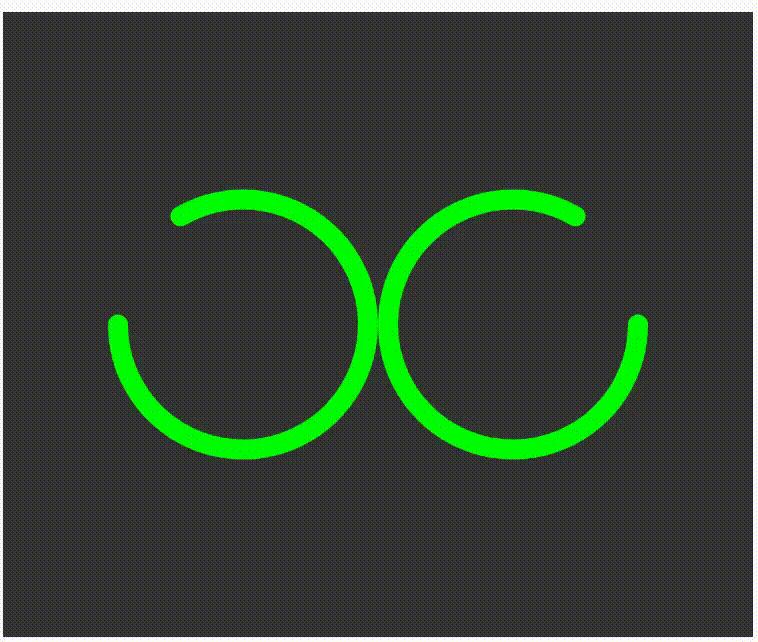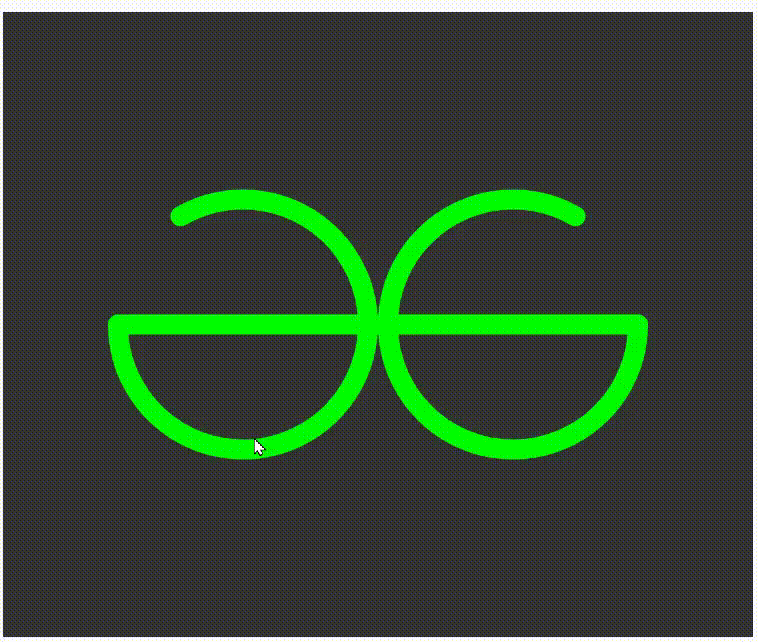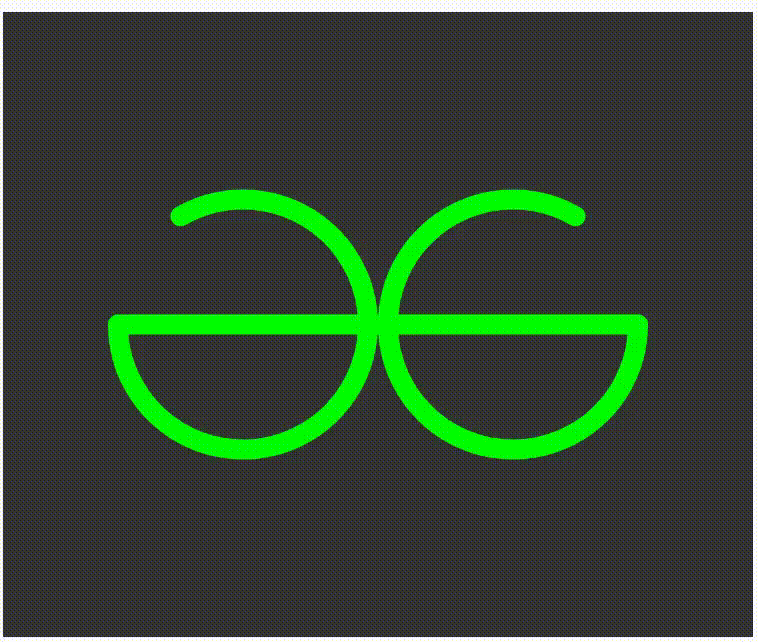Open in App
Not now

# How to create GeeksforGeeks logo using p5.js ?

• Last Updated : 18 Jan, 2023

In this article, we will see how to create a GeeksforGeeks logo using p5.js.

Processing is a flexible software sketchbook and a language for learning how to code within the context of the visual arts. We can create different types of arts using our coding skills example is like games, animation And physics engines, etc.

Approach:

• Set the function setup() which put output window size.
• Initialize a variable with random value(offset = 108).
• Set background colour,nofill, stroke, and position of the logo in draw() function.
• Then start to draw the logo:
• Create two arcs inverted  ‘C’ shape.
• Create two horizontal lines in mid of the arcs.
• Make the center of the arcs zero.
• Our logo is complete.

Below is the implementation of above approach.

Step 1: Create two arcs as shown below:

## Javascript

 `// Create arc``arc(width/2 - offset, height/2, 200, 200, -PI + PI/3, PI);``arc(width/2 + offset, height/2, 200, 200, 0, 2*PI - PI/3);`

Output:Step 2: Create Horizontal lines

## Javascript

 `// Horizontal lines``line(width/2 + offset + 100*sin(PI/2), height/2, width/2, height/2);``line(width/2 - offset - 100*sin(PI/2), height/2, width/2, height/2);`Example:

## Javascript

 `// Create a variable.` `var` `offset;``function` `setup() {``  ``// Set the size of output window.``  ``createCanvas(600, 500);``  ` `  ``// Set the value of offset``  ``offset = 108;``}` `function` `draw() {``  ` `  ``// Set the background colour.``  ``background(51);``  ``noFill();``  ``stroke(0, 255, 0);``  ``strokeWeight(16);``  ` `  ``// Set the ellipse mode in center.``  ``ellipseMode(CENTER);``  ` `  ``// Arc of both sides.``  ``arc(width/2 - offset, height/2, 200, 200, -PI + PI/3, PI);``  ``arc(width/2 + offset, height/2, 200, 200, 0, 2*PI - PI/3);``  ` `  ``// Horizontal lines``  ``line(width/2 + offset + 100*sin(PI/2), height/2, width/2, height/2);``  ``line(width/2 - offset - 100*sin(PI/2), height/2, width/2, height/2);``  ``push();``  ` `  ``// Make the value of center zero.``  ``translate(width/2 - offset, height/2);``  ` `  ` `  ``pop();``  ``push();``  ` `  ``// Make the value of center zero.``  ``translate(width/2 + offset, height/2);``  ` `  ` `  ``pop();``}`

Output:My Personal Notes arrow_drop_up